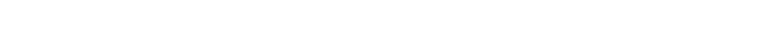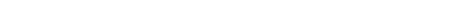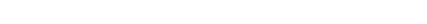Learn this powerful math trick for GMAT Problem Solving questions.

First, try these two practice GMAT Quantitative section Problem Solving questions.

1) Bert left the house with N dollars.  He spent 1/4 of this at the hardware store, then \$7 at the dry cleaners, and then half of what was left at the grocery store.  When he got home, he had \$10 left in his pocket.  What was the value of N?

(A) \$36
(B) \$44
(C) \$52
(D) \$60
(E) \$68

2) Ms. Morris invested in Fund A and Fund B.  The total amount he invested, in both funds combined, was \$100,000. In one year, Fund A paid 23% and Fund B paid 17%.  The interest earned in Fund B was exactly \$200 greater than the interest earned in Fund A.  How much did Ms. Morris invest in Fund A?

(A) \$32,000
(B) \$36,000
(C) \$40,000
(D) \$42,000
(E) \$45,000(A) 13
(B) 22
(C) 33
(D) 46
(E) 78

A powerful math trick

Do you notice what all three of these Problem Solving questions have in common?  In all three, the five answer choices are numerical.  Whenever an unknown is given in a word problem, and the answer choices are possible numerical values for this unknown, you can plug in.

What does it mean to plug in (also known as “solution behavior“).

Of course, if time is not a huge issue, you just repeat the process.  If (C) is too small, try either (D) or (E) next.  If (C) is too big, then try (A) or (B) next.  By the end of the second plug in, you should know exactly what the answer is.

As an example, I will solve sample problem #1.  This one could be solved with a variety of mean, but to demonstrate the strategy, I will employ backsolving.  Start with answer (C): let’s say that N = 52.  Well, first Fred spends 1/4, that is \$13, at the hardware store, which leaves him with \$39 dollars.  Then, he spends \$7 at the dry cleaners, leaving him with \$32.  Then he spends half at the grocery store, which leave him with \$16.  This is more than the \$10 which he is supposed to have at the end of his trip.  (C) led to a value that is too large, so right away, we know, (C) & (D) & (E) are all wrong.  Now, try (B): let’s say N = \$44.  Well, first Fred spends 1/4, that is \$11, at the hardware store, which leaves him with \$33 dollars.  Then, he spends \$7 at the dry cleaners, leaving him with \$26.  Then he spends half at the grocery store, which leave him with \$13.  This is still more than \$10.  (B) is also too big.  This can only mean that (A) is the answer.  Two plug ins, and you are done.  If you were toward the end of the Quantitative section, and had oceans of time left, you could take the extra step of double-checking by backsolving with (A) to verify, but only do that extra step if you really feel that you have more than enough time remaining.

More practice

Now that you’ve read this section, give those other two problems a try with plugging in, before reading the solutions below.  Here’s another Magoosh problem on which you can also practice:

Practice problem solutions

1) A

2) This one could be solved with a good deal of hairy algebra, but backsolving is an incredibly efficient alternative.  Let’s start with (C) — we’ll say Ms. Morris invested \$40,000 in Fund A, which means she invested \$60,0000 in fund B.  In Fund A, she earned 23%, which was (0.23)*40000 = \$9200.  In Fund B, she earned 17%, which was (0.17)*60000 = \$10200.  Here, the interest earned in Fund B was \$1000 more than the interest earned in Fund A, a bigger difference than we want.  To make the difference smaller, we need less money in Fund B and more money in Fund A.  Eliminate answers (A) & (B) & (C).  Try D: We’ll say Ms. Morris invested \$42,000 in Fund A, which means she invested \$58,000 in Fund B.  In Fund A, she earned 23%, which was (0.23)*42000 = \$9660.  In Fund B, she earned 17%, which was (0.17)*58000 = \$9860.  The difference is exactly \$200, so D is the answer.

3) For this one, a frontal assault with algebra is likely to be an unproductive disaster.  Backsolving is really the way to go.  Notice that each of the three terms increases as n increase, so we have reason to believe the value of a(n) increase as n increases.  Let’s start with n= 33.  We have to do a little arithmetic to figure out that 33^2 = 1089, but then we can calculate thatThat’s much bigger than 500.  Presumably, the very first term larger than 500 would not be that much bigger than 500.  Let’s go down one, to (B).  Suppose n = 22.  Again, we have to do a little arithmetic to calculate that 22^2 = 484, but then we can compute:Aha!  This is just over 500, and the answers are spaced out enough, that the next one, (A), will undoubtedly be much smaller.  Therefore, answer = B.

Author

•Mike served as a GMAT Expert at Magoosh, helping create hundreds of lesson videos and practice questions to help guide GMAT students to success. He was also featured as "member of the month" for over two years at GMAT Club. Mike holds an A.B. in Physics (graduating magna cum laude) and an M.T.S. in Religions of the World, both from Harvard. Beyond standardized testing, Mike has over 20 years of both private and public high school teaching experience specializing in math and physics. In his free time, Mike likes smashing foosballs into orbit, and despite having no obvious cranial deficiency, he insists on rooting for the NY Mets. Learn more about the GMAT through Mike's Youtube video explanations and resources like What is a Good GMAT Score? and the GMAT Diagnostic Test.